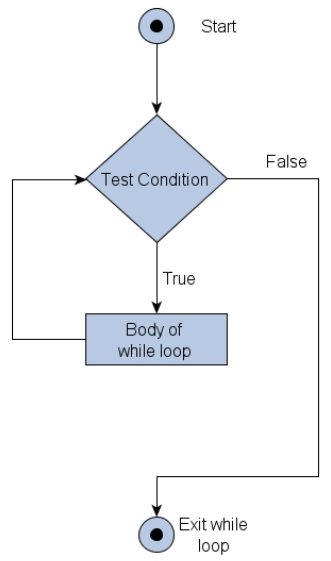×

Search anything:

# While loop in C

#### C Programming Software Engineering while loopGet this book -> Problems on Array: For Interviews and Competitive Programming

A loop is used for executing a block of statements repeatedly until a given condition returns false. These loops save the programmer from writing long lines of codes performing the same task over and over again. C provides three kinds of loops:

• for loop
• while loop
• do-while loop

## Parts of a loop

Loops consist of four major elements that govern the execution and working of a loop:

1. Initialization Expression(s) The initialization of the control variable takes place under this.
2. Test Expression The expression whose truth value decides whether the loop-body will get executed or not.
• In an entry-controlled loop the test expression is evaluated before entering into a loop.
• In an exit-controlled loop the test expression is evaluated before exiting from the loop.
1. Update Expression(s) These change the value of loop variable(s).

2. The Body of the loop The statements that are executed repeatedly form the body of the loop.

## While loop

SYNTAX

``````while(condition){

//Statements to be executed repeatedly
// Increment (++) or Decrement (--) Operation
}
``````

FLOW DIAGRAM## Order of execution

1. The control variable is initialized.
2. The test expression is evaluated
3. If it evaluates to true, the loop enters the body
4. And it is repeatedly executed, till the expression does not evaluate to false
5. When the test expression evaluates to false, the loop is exited.

Example:

``````#include <stdio.h>
int main()
{
int i=0;
while(i<=2){
printf("Hello\n");
i=i+1;
}
printf("Out of loop");
}
``````

Output:

``````Hello
Hello
Hello
Out of loop
``````

## `while` Vs `do while` loop

1. Syntax

Syntax of while loop as follows:

``````while(condition)
{
//statements;
}
``````

Syntax of do while loop:

``````do
{
//statements;
} wile(condition);
``````

2. Loop type

While loop is an entry controlled loop while do while loop is an exit controlled loop

3. Iterations

If condition is false, then no iteration take place in while loop but in case of do while loop, one iteration take place irrespective of the condition.

4. Example

Example of while loop:

``````int i=0,sum=0;
while(i<=10)
{
sum = sum + i;
i++;
}
``````

Example of do while loop:

``````int i=0, sum=0;
do
{
sum = sum + i;
i++;
} while(i<=10);
``````

#### Application

• Used when a condition needs to be fulfilled in order for a process to work.
• The condition needs to be checked before the process starts.
• Unlike a do-while loop, the body of the loop won't get executed until and unless the condition evaluates to true.

## Question

#### while(1) means what?

infinite loop
work till 1 comes
till the variable is equal to 1
none
While loop works only till the test condition evaluates to true, and any number except 0 implies true. Therefore while(1) will always evaluate to true, and hence the loop will keep on working infinitely.